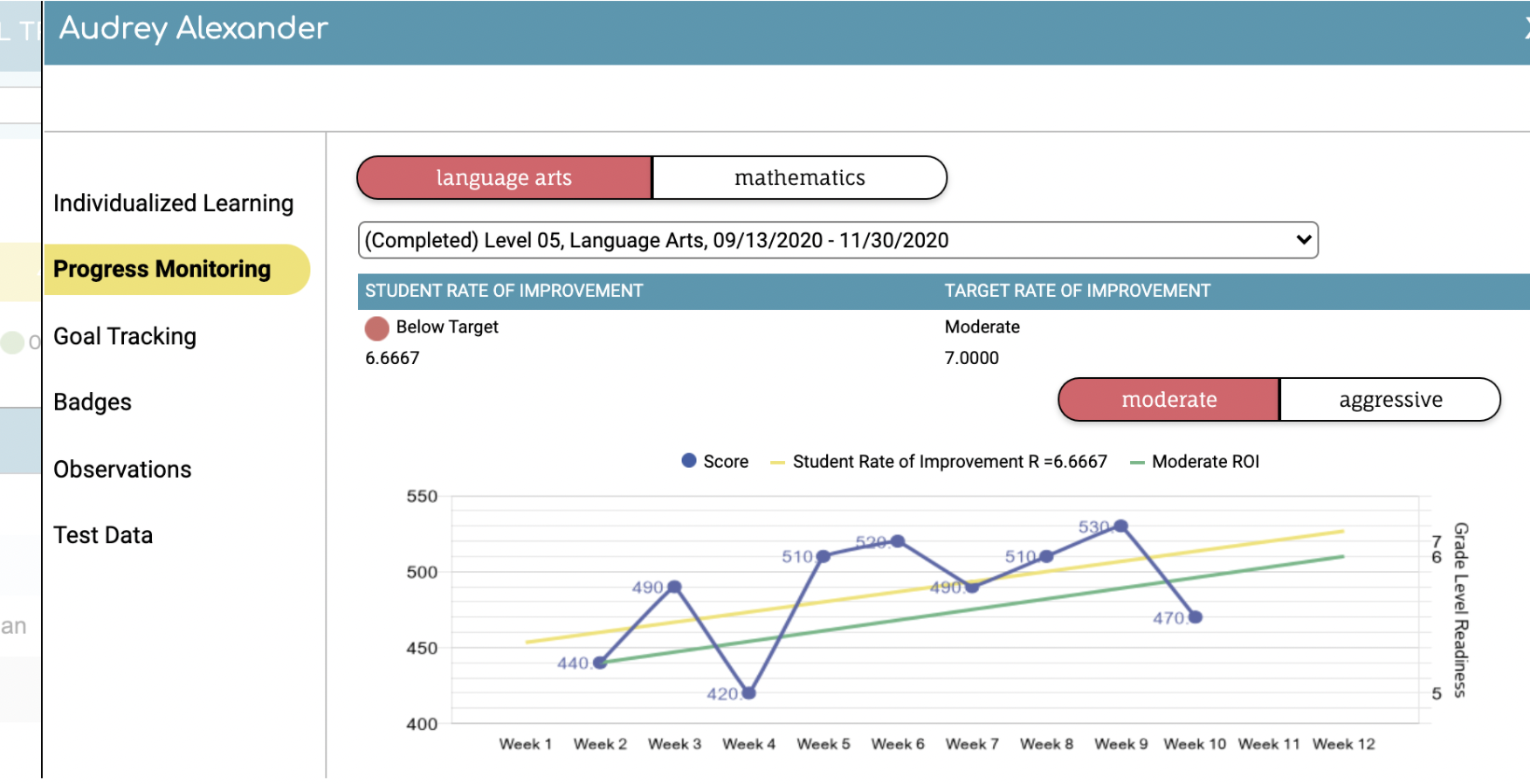# How is the student's Current ROI Calculated for Curriculum Sampling Probes?

The student's Current ROI, or Rate of Improvement is an indicator to how well the student is responding to their intervention. This can also be referred to as a trend line. This trend line is a prediction of how a student will progress during the intervention period based on their CBM results. This is initially calculated after three probes have been completed. On the graph, Classworks calculates the trend line using an ordinary least squares regression equation. In essence, it comes down to the number of points the student is expected to improve each week.As the student takes additional probes, Classworks uses the new scores to adjust the trend line and improve the accuracy of the prediction.
• On the graph, the yellow line indicates the student's Current ROI (trend line).

• The Current ROI line will only display after the student has completed at least 3 CBMs.

If the student's current ROI indicates a downward trend or if the student's current ROI is indicating that the student is trending towards not making acceptable progress, we recommend the student support team make changes to the intervention.